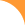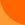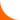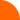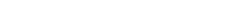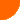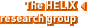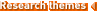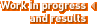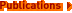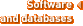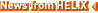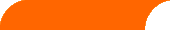Publications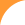On Metric Generators of Graphs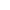Mathematics of Operations Research. 2004 May. 29(2 ):383-393A. Sebö, E. Tannier
 Abstract: We study generators of metric spaces, that is sets of points with the property that every point of the space is uniquely determined by the distances from their elements. Such generators put a light on seemingly different kinds of problems in combinatorics that are not directly related to metric spaces. The two applications we present concern combinatorial search --- problems on false coins known from the borderline of extremal combinatorics and information theory ---, and a problem known from combinatorial optimization: connected joins in graphs. We use results on the detection of false coins to approximate the metric dimension (minimum size of a generator for the metric space defined by the distances) of some particular graphs for which the problem was known and open. In the opposite direction, using metric generators, we show that the existence of connected joins in graphs can be solved in polynomial time, a problem asked in a survey paper of Frank. On the negative side we prove that the minimization of the number of components of a join is NP-hard. We further explore the metric dimension with some problems. The main problem we are led to is how to extend an isometry given on a metric generator of a metric space.